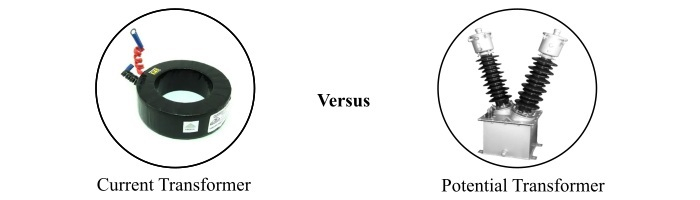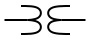# Difference between Current Transformer and Potential Transformer

A transformer is a static electrical machine that is used for increasing or decreasing the value of alternating voltage or current. Based on the applications, the transformers are classified into following main types −

• Power transformer

• Distribution transformer

• Instrument transformer

The instrument transformers are used for the protection and measurement purposes in the electrical power systems and are further classified into two types viz. current transformer (CT) and potential transformer (PT).In this article, we will explain the differences between current transformer and potential transformer along with their basic description.

## What is a Current Transformer?

A current transformer (CT) is a type of transformer that is mainly designed to reduce the high currents to a lower value for measurement and protection purposes. Basically a current transformer is a voltage step-up and current step-down transformer that means when it reduces the current, increases the voltage in the same ratio.

As in an electrical power system, the current at which transmission and distribution lines operate is very high, but the protecting devices such as relays, circuit breaker controllers, contactors and the measuring instruments like ammeters, voltmeters, watt-meters, energy meters, etc. require a very small current to function. Hence, the high current from the transmission and distribution lines is reduced to a desired value before suppling it to instruments by employing a current transformer.

The current transformer consists of a primary winding which has less number of turns, usually one turn, and a secondary winding having more number turns depending on the CT ratio. The primary winding of the current transformer is directly connected to the line whose current is to be measured, while the secondary winding is connected to the ammeter or any other device.

## What is a Potential Transformer?

A potential transformer (PT), also known as voltage transformer (VT), is another type of instrument transformer which is designed to reduce the high voltage of a power line to a lower voltage for measurement and protection applications.

The potential transformer consists of a primary winding of more number of turns and a secondary winding with less number of turs depending on the PT ratio. The primary winding is connected to the power line whose voltage need to be measured while the secondary winding is connected to a voltmeter to show the reading of the voltage.

## Difference between Current Transformer and Potential Transformer

Both current and potential transformers are types of instrument transformer used for measurement and protection in an electrical power system. However, there are many differences between CT and PT that are highlighted in the following table −

Basis of Difference Current Transformer Potential Transformer
Definition An instrument transformer that is used to reduce high currents of power lines to the lower values for measurement is called a current transformer. An instrument transformer that is used to reduce the high voltages of power lines to the low voltages for measurement is called a potential transformer.
Abbreviated name CT is used as the abbreviated name to represent a current transformer. PT (or sometimes VT) is used to denote a potential transformer.
SymbolFunction CT reduces the large current to a safer and measurable level. PT reduces a high voltage to a safer and measurable level.
Step up/down Current transformer is a voltage step-up and current step-down transformer. Potential transformer is a voltage step-down and current step-up transformer.
Types The main types of current transformers are − Wound Types CT, Toroidal CT, Bar Type CT and Summation CT. The two major types of potential transformers are − Electromagnetic PT and Capacitive PT.
Number of turns In a current transformer, the number of turns in primary winding are less than that are in the secondary winding. Usually only one turn is provided in the primary winding of CT. In a potential transformer, the primary winding has more turns while secondary winding has less number of turns.
Connection of primary winding The primary winding of a current transformer is connected in series with a power line whose current is to be measured. The primary winding of a potential transformer is connected in parallel with a power line whose voltage is to be measured.
Transformation ratio Current transformer has a high transformation ratio, and commonly known as CT ratio. Potential transformer has a low transformation ratio that is known as PT ratio.
Secondary winding open In case of current transformer, the secondary winding can never be open while operating, it may damage the whole transformer and cause an accident. The secondary winding of a potential transformer can be open while operating.
Conductor size In a current transformer, the primary winding conductor is thicker to carry high currents. The secondary winding conductor is thinner as it carries low currents. In a PT, the conductor used for primary winding is thin, while the secondary conductor is thick.
Secondary winding rating Industrial standards have established 1 A or 5 A as the secondary current rating of a current transformer. Industrial standards have established 110 volts as the secondary voltage rating of a potential transformer.
Effect of burden on accuracy The accuracy of a current transformer does not depend on the burden on CT, i.e. we can connected any number of ammeters to the secondary winding The accuracy of the potential transformer depends on the burden that means if numerous voltmeters are connected with the secondary, it may cause an error.
Core material The core of current transformer is constructed by silicon steel laminations. The core of potential transformer is constructed with high quality steel so it can operate at low flux densities.
Applications Current transformers are mainly used to measure the high currents using usual ammeters and also used for operating protective devices such as relays and contactors. PT is primarily used for measuring the voltages of electric power lines.

## Conclusion

The most significant difference between a current transformer (CT) and a potential transformer (PT) is that the current transformer is a step-up transformer while the potential transformer is a step-down transformer. However, both CT and PT are the instrument transformers used for measurement and protection purposes in an electric power system.